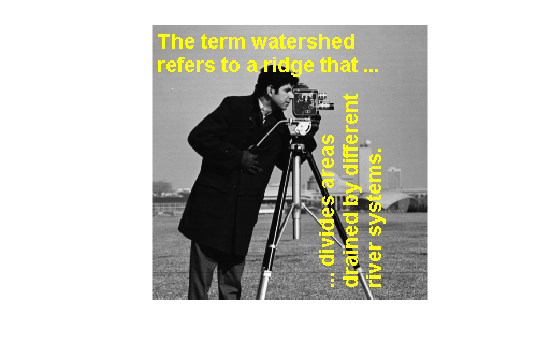# imoverlay

Burn binary mask into 2-D image

## Syntax

``B = imoverlay(A,BW)``
``B = imoverlay(A,BW,color)``

## Description

example

````B = imoverlay(A,BW)` fills the grayscale or RGB image `A` with a solid color where the input binary mask, `BW`, is `true`.```
````B = imoverlay(A,BW,color)` specifies the color that `imoverlay` uses to fill the image.```

## Examples

collapse all

Read a grayscale image into the workspace.

`A = imread('cameraman.tif');`

Read a binary image into the workspace.

`BW = imread('text.png');`

Burn the binary image into the grayscale image, specifying the color to be used for the binary mask.

`B = imoverlay(A,BW,'yellow');`

Display the result.

`imshow(B)`Read an RGB image into the workspace.

`RGB = imread('peppers.png');`

Read a binary image into the workspace.

`BW = imread('text.png');`

Crop the RGB image to make it the same size as the binary mask.

`RGB_cropped = imcrop(RGB,[64,128,255,255]);`

Burn the binary image into the cropped RGB image, choosing the color to be used.

`B = imoverlay(RGB_cropped,BW,'red');`

Display the result.

```figure imshow(B)```## Input Arguments

collapse all

Input image, specified as a 2-D grayscale image or 2-D RGB image.

Data Types: `single` | `double` | `int16` | `uint8` | `uint16` | `logical`

Mask image, specified 2-D binary matrix of the same size as the first two dimensions of image `A`. For numeric input, any nonzero pixels are considered to be `1` (`true`).

Data Types: `single` | `double` | `int8` | `int16` | `int32` | `uint8` | `uint16` | `uint32` | `logical`

Color used for the overlay, specified as a MATLAB color specification. For example, if you want to specify the color red, you could use any of the following specifications: `'red'`, `'r'`, or `[1 0 0]`.

## Output Arguments

collapse all

Output image, returned as a 2-D RGB image.

Data Types: `uint8`